Anzeige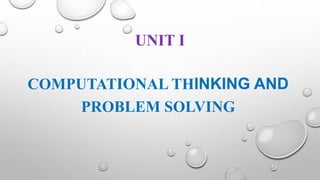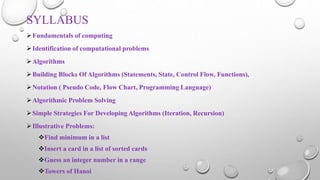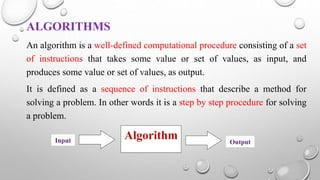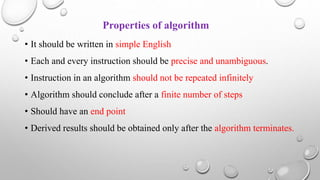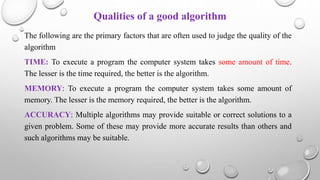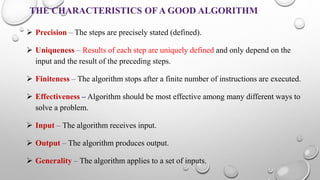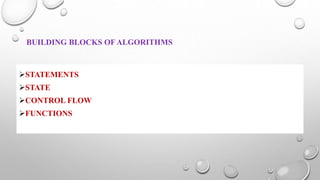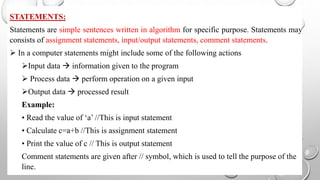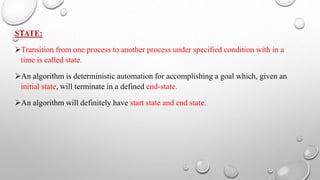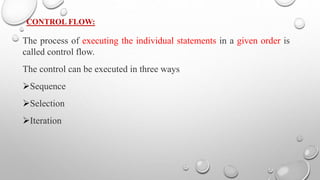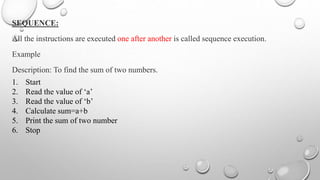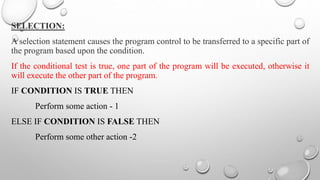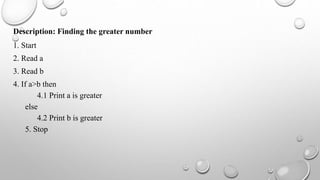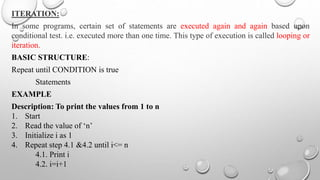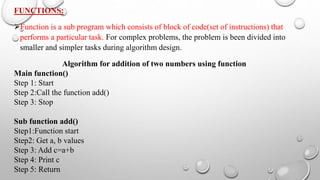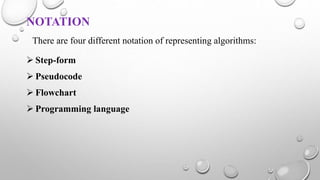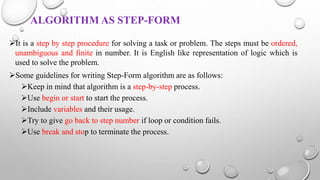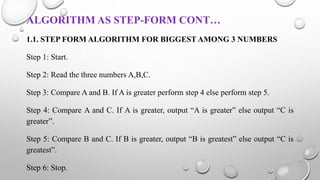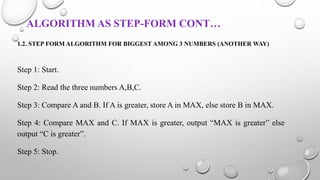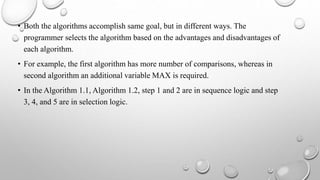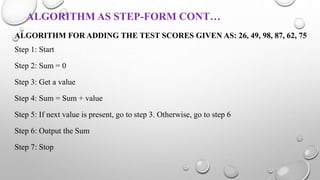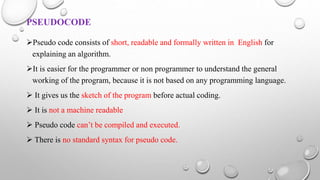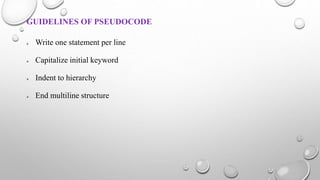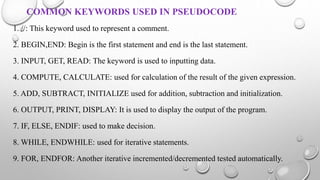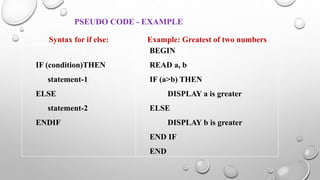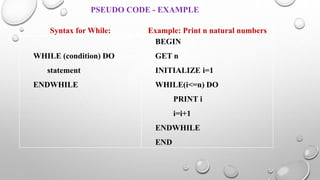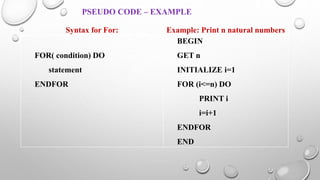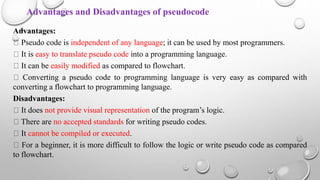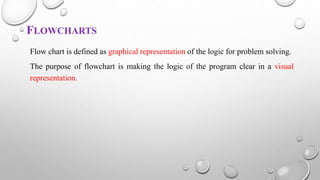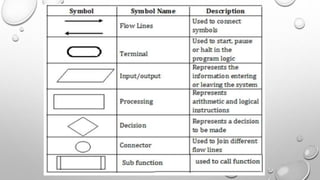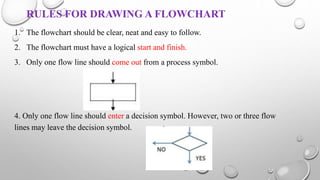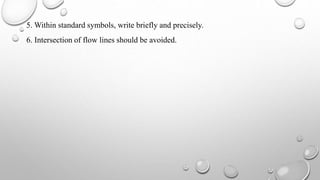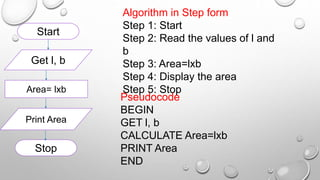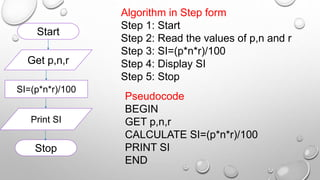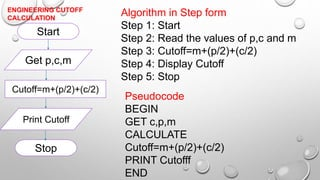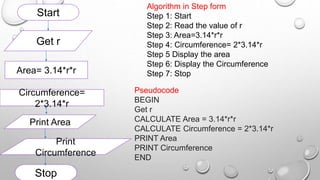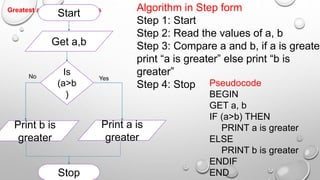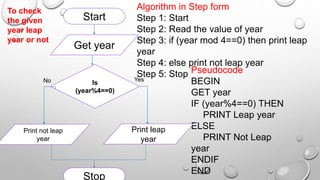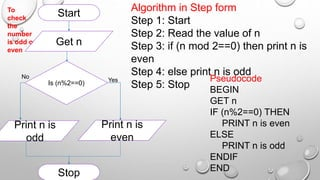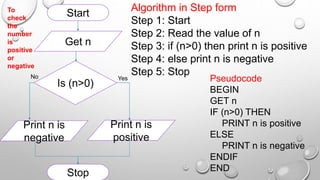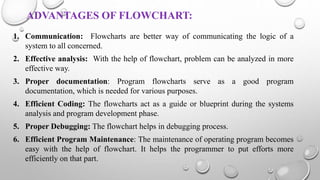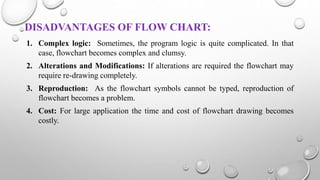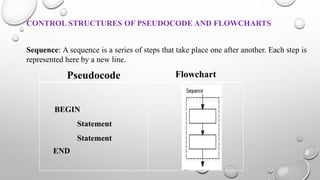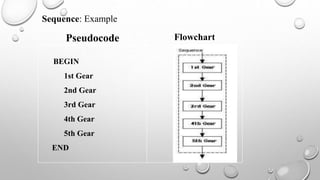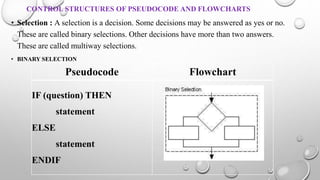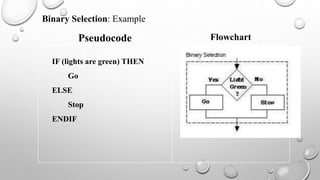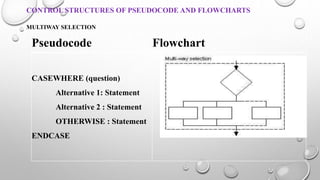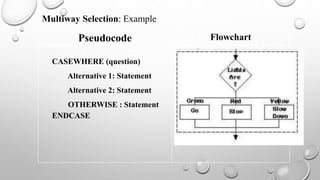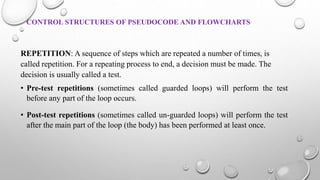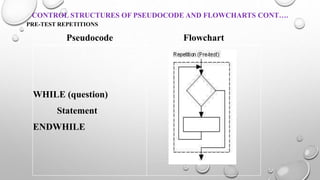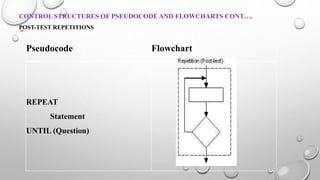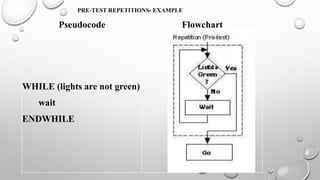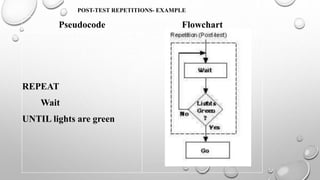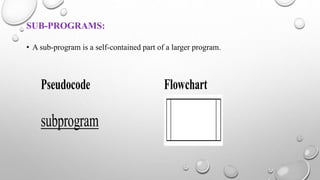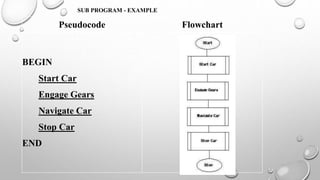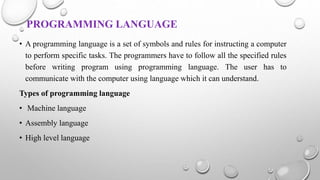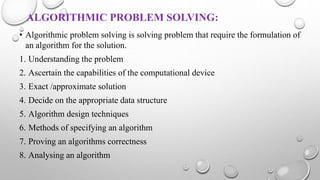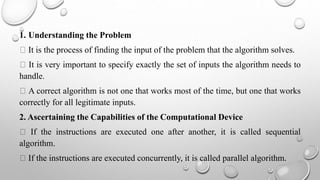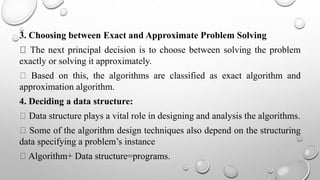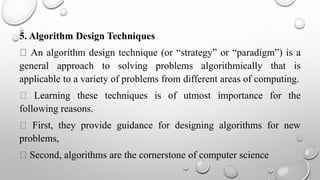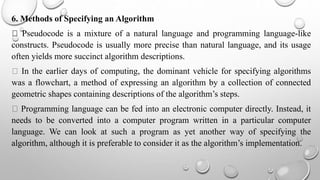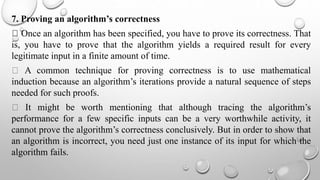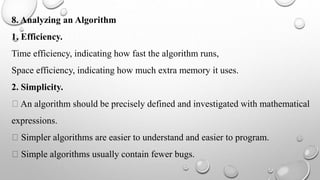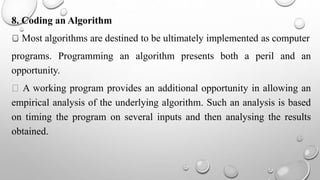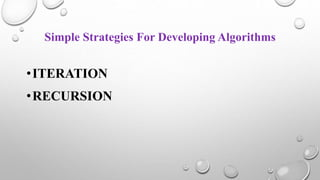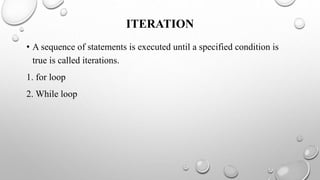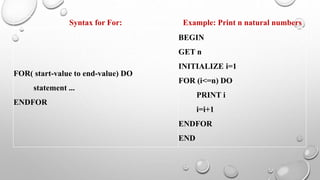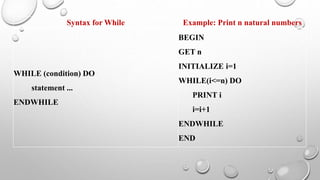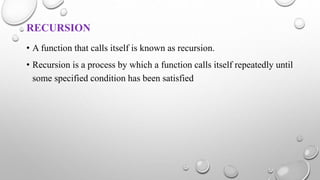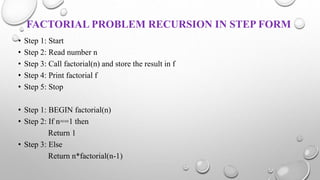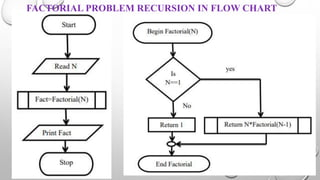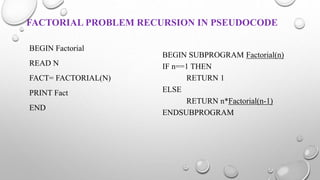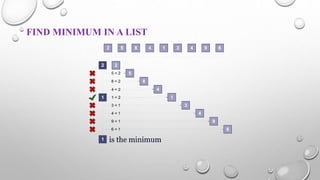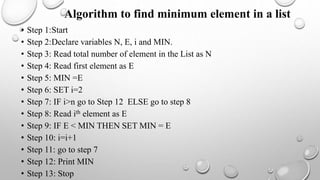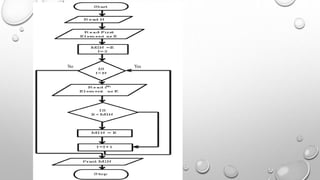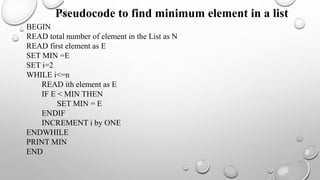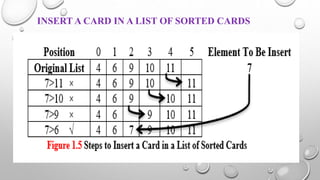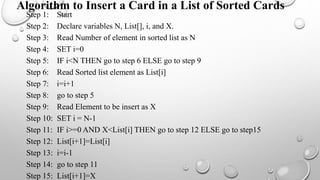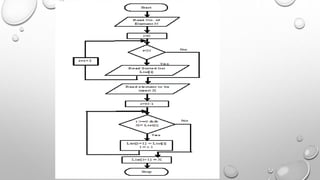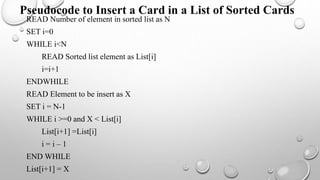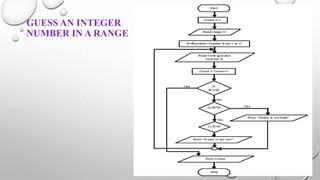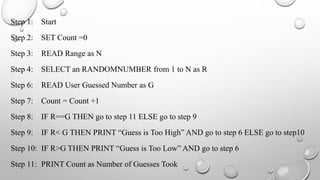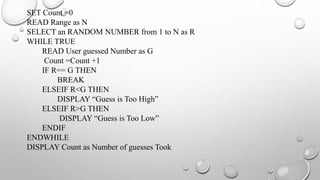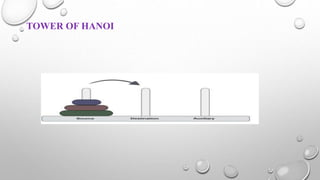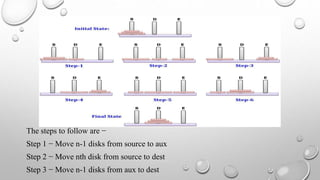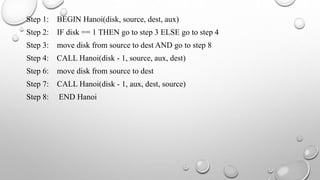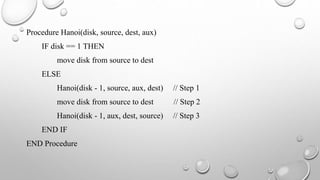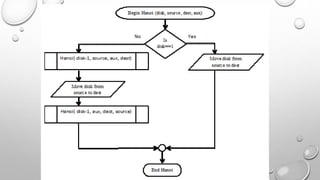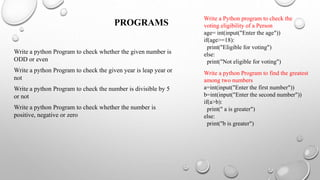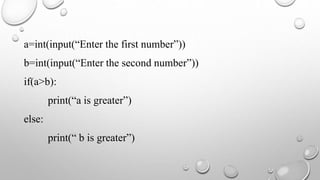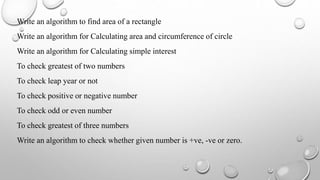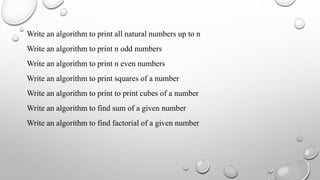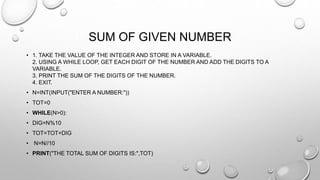1 von 94
Anzeige

### UNIT 1.pptx

1. UNIT I COMPUTATIONAL THINKING AND PROBLEM SOLVING
2. SYLLABUS Fundamentals of computing Identification of computational problems Algorithms Building Blocks Of Algorithms (Statements, State, Control Flow, Functions), Notation ( Pseudo Code, Flow Chart, Programming Language) Algorithmic Problem Solving Simple Strategies For Developing Algorithms (Iteration, Recursion) Illustrative Problems: Find minimum in a list Insert a card in a list of sorted cards Guess an integer number in a range Towers of Hanoi
3. ALGORITHMS An algorithm is a well-defined computational procedure consisting of a set of instructions that takes some value or set of values, as input, and produces some value or set of values, as output. It is defined as a sequence of instructions that describe a method for solving a problem. In other words it is a step by step procedure for solving a problem. Algorithm Input Output
4. • It should be written in simple English • Each and every instruction should be precise and unambiguous. • Instruction in an algorithm should not be repeated infinitely • Algorithm should conclude after a finite number of steps • Should have an end point • Derived results should be obtained only after the algorithm terminates. Properties of algorithm
5. Qualities of a good algorithm The following are the primary factors that are often used to judge the quality of the algorithm TIME: To execute a program the computer system takes some amount of time. The lesser is the time required, the better is the algorithm. MEMORY: To execute a program the computer system takes some amount of memory. The lesser is the memory required, the better is the algorithm. ACCURACY: Multiple algorithms may provide suitable or correct solutions to a given problem. Some of these may provide more accurate results than others and such algorithms may be suitable.
6. THE CHARACTERISTICS OF A GOOD ALGORITHM  Precision – The steps are precisely stated (defined).  Uniqueness – Results of each step are uniquely defined and only depend on the input and the result of the preceding steps.  Finiteness – The algorithm stops after a finite number of instructions are executed.  Effectiveness – Algorithm should be most effective among many different ways to solve a problem.  Input – The algorithm receives input.  Output – The algorithm produces output.  Generality – The algorithm applies to a set of inputs.
7. BUILDING BLOCKS OF ALGORITHMS STATEMENTS STATE CONTROL FLOW FUNCTIONS
8. STATEMENTS: Statements are simple sentences written in algorithm for specific purpose. Statements may consists of assignment statements, input/output statements, comment statements.  In a computer statements might include some of the following actions Input data  information given to the program  Process data  perform operation on a given input Output data  processed result Example: • Read the value of ‘a’ //This is input statement • Calculate c=a+b //This is assignment statement • Print the value of c // This is output statement Comment statements are given after // symbol, which is used to tell the purpose of the line.
9. STATE: Transition from one process to another process under specified condition with in a time is called state. An algorithm is deterministic automation for accomplishing a goal which, given an initial state, will terminate in a defined end-state. An algorithm will definitely have start state and end state.
10. CONTROL FLOW: The process of executing the individual statements in a given order is called control flow. The control can be executed in three ways Sequence Selection Iteration
11. SEQUENCE: All the instructions are executed one after another is called sequence execution. Example Description: To find the sum of two numbers. 1. Start 2. Read the value of ‘a’ 3. Read the value of ‘b’ 4. Calculate sum=a+b 5. Print the sum of two number 6. Stop
12. SELECTION: A selection statement causes the program control to be transferred to a specific part of the program based upon the condition. If the conditional test is true, one part of the program will be executed, otherwise it will execute the other part of the program. IF CONDITION IS TRUE THEN Perform some action - 1 ELSE IF CONDITION IS FALSE THEN Perform some other action -2
13. Description: Finding the greater number 1. Start 2. Read a 3. Read b 4. If a>b then 4.1 Print a is greater else 4.2 Print b is greater 5. Stop
14. ITERATION: In some programs, certain set of statements are executed again and again based upon conditional test. i.e. executed more than one time. This type of execution is called looping or iteration. BASIC STRUCTURE: Repeat until CONDITION is true Statements EXAMPLE Description: To print the values from 1 to n 1. Start 2. Read the value of ‘n’ 3. Initialize i as 1 4. Repeat step 4.1 &4.2 until i<= n 4.1. Print i 4.2. i=i+1
15. FUNCTIONS: Function is a sub program which consists of block of code(set of instructions) that performs a particular task. For complex problems, the problem is been divided into smaller and simpler tasks during algorithm design. Algorithm for addition of two numbers using function Main function() Step 1: Start Step 2:Call the function add() Step 3: Stop Sub function add() Step1:Function start Step2: Get a, b values Step 3: Add c=a+b Step 4: Print c Step 5: Return
16. NOTATION There are four different notation of representing algorithms:  Step-form  Pseudocode  Flowchart  Programming language
17. ALGORITHM AS STEP-FORM It is a step by step procedure for solving a task or problem. The steps must be ordered, unambiguous and finite in number. It is English like representation of logic which is used to solve the problem. Some guidelines for writing Step-Form algorithm are as follows: Keep in mind that algorithm is a step-by-step process. Use begin or start to start the process. Include variables and their usage. Try to give go back to step number if loop or condition fails. Use break and stop to terminate the process.
18. ALGORITHM AS STEP-FORM CONT… 1.1. STEP FORM ALGORITHM FOR BIGGEST AMONG 3 NUMBERS Step 1: Start. Step 2: Read the three numbers A,B,C. Step 3: Compare A and B. If A is greater perform step 4 else perform step 5. Step 4: Compare A and C. If A is greater, output “A is greater” else output “C is greater”. Step 5: Compare B and C. If B is greater, output “B is greatest” else output “C is greatest”. Step 6: Stop.
19. ALGORITHM AS STEP-FORM CONT… 1.2. STEP FORM ALGORITHM FOR BIGGEST AMONG 3 NUMBERS (ANOTHER WAY) Step 1: Start. Step 2: Read the three numbers A,B,C. Step 3: Compare A and B. If A is greater, store A in MAX, else store B in MAX. Step 4: Compare MAX and C. If MAX is greater, output “MAX is greater” else output “C is greater”. Step 5: Stop.
20. • Both the algorithms accomplish same goal, but in different ways. The programmer selects the algorithm based on the advantages and disadvantages of each algorithm. • For example, the first algorithm has more number of comparisons, whereas in second algorithm an additional variable MAX is required. • In the Algorithm 1.1, Algorithm 1.2, step 1 and 2 are in sequence logic and step 3, 4, and 5 are in selection logic.
21. ALGORITHM AS STEP-FORM CONT… ALGORITHM FOR ADDING THE TEST SCORES GIVEN AS: 26, 49, 98, 87, 62, 75 Step 1: Start Step 2: Sum = 0 Step 3: Get a value Step 4: Sum = Sum + value Step 5: If next value is present, go to step 3. Otherwise, go to step 6 Step 6: Output the Sum Step 7: Stop
22. PSEUDOCODE Pseudo code consists of short, readable and formally written in English for explaining an algorithm. It is easier for the programmer or non programmer to understand the general working of the program, because it is not based on any programming language.  It gives us the sketch of the program before actual coding.  It is not a machine readable  Pseudo code can’t be compiled and executed.  There is no standard syntax for pseudo code.
23. GUIDELINES OF PSEUDOCODE  Write one statement per line  Capitalize initial keyword  Indent to hierarchy  End multiline structure
24. COMMON KEYWORDS USED IN PSEUDOCODE 1. //: This keyword used to represent a comment. 2. BEGIN,END: Begin is the first statement and end is the last statement. 3. INPUT, GET, READ: The keyword is used to inputting data. 4. COMPUTE, CALCULATE: used for calculation of the result of the given expression. 5. ADD, SUBTRACT, INITIALIZE used for addition, subtraction and initialization. 6. OUTPUT, PRINT, DISPLAY: It is used to display the output of the program. 7. IF, ELSE, ENDIF: used to make decision. 8. WHILE, ENDWHILE: used for iterative statements. 9. FOR, ENDFOR: Another iterative incremented/decremented tested automatically.
25. Syntax for if else: Example: Greatest of two numbers IF (condition)THEN statement-1 ELSE statement-2 ENDIF BEGIN READ a, b IF (a>b) THEN DISPLAY a is greater ELSE DISPLAY b is greater END IF END PSEUDO CODE - EXAMPLE
26. Syntax for While: Example: Print n natural numbers WHILE (condition) DO statement ENDWHILE BEGIN GET n INITIALIZE i=1 WHILE(i<=n) DO PRINT i i=i+1 ENDWHILE END PSEUDO CODE - EXAMPLE
27. Syntax for For: Example: Print n natural numbers FOR( condition) DO statement ENDFOR BEGIN GET n INITIALIZE i=1 FOR (i<=n) DO PRINT i i=i+1 ENDFOR END PSEUDO CODE – EXAMPLE
28. Advantages and Disadvantages of pseudocode Advantages: Pseudo code is independent of any language; it can be used by most programmers. It is easy to translate pseudo code into a programming language. It can be easily modified as compared to flowchart. Converting a pseudo code to programming language is very easy as compared with converting a flowchart to programming language. Disadvantages: It does not provide visual representation of the program’s logic. There are no accepted standards for writing pseudo codes. It cannot be compiled or executed. For a beginner, it is more difficult to follow the logic or write pseudo code as compared to flowchart.
29. FLOWCHARTS Flow chart is defined as graphical representation of the logic for problem solving. The purpose of flowchart is making the logic of the program clear in a visual representation.
30. RULES FOR DRAWING A FLOWCHART 1. The flowchart should be clear, neat and easy to follow. 2. The flowchart must have a logical start and finish. 3. Only one flow line should come out from a process symbol. 4. Only one flow line should enter a decision symbol. However, two or three flow lines may leave the decision symbol.
31. 5. Within standard symbols, write briefly and precisely. 6. Intersection of flow lines should be avoided.
32. Start Get l, b Area= lxb Print Area Stop Algorithm in Step form Step 1: Start Step 2: Read the values of l and b Step 3: Area=lxb Step 4: Display the area Step 5: Stop Pseudocode BEGIN GET l, b CALCULATE Area=lxb PRINT Area END
33. Start Get p,n,r SI=(p*n*r)/100 Print SI Stop Algorithm in Step form Step 1: Start Step 2: Read the values of p,n and r Step 3: SI=(p*n*r)/100 Step 4: Display SI Step 5: Stop Pseudocode BEGIN GET p,n,r CALCULATE SI=(p*n*r)/100 PRINT SI END
34. Start Get p,c,m Cutoff=m+(p/2)+(c/2) Print Cutoff Stop Algorithm in Step form Step 1: Start Step 2: Read the values of p,c and m Step 3: Cutoff=m+(p/2)+(c/2) Step 4: Display Cutoff Step 5: Stop Pseudocode BEGIN GET c,p,m CALCULATE Cutoff=m+(p/2)+(c/2) PRINT Cutofff END ENGINEERING CUTOFF CALCULATION
35. Start Get r Area= 3.14*r*r Print Area Stop Circumference= 2*3.14*r Print Circumference Algorithm in Step form Step 1: Start Step 2: Read the value of r Step 3: Area=3.14*r*r Step 4: Circumference= 2*3.14*r Step 5 Display the area Step 6: Display the Circumference Step 7: Stop Pseudocode BEGIN Get r CALCULATE Area = 3.14*r*r CALCULATE Circumference = 2*3.14*r PRINT Area PRINT Circumference END
36. Algorithm in Step form Step 1: Start Step 2: Read the values of a, b Step 3: Compare a and b, if a is greater print “a is greater” else print “b is greater” Step 4: Stop Pseudocode BEGIN GET a, b IF (a>b) THEN PRINT a is greater ELSE PRINT b is greater ENDIF END Greatest among two numbers Yes Start Get a,b Is (a>b ) Print a is greater Print b is greater No Stop
37. Algorithm in Step form Step 1: Start Step 2: Read the value of year Step 3: if (year mod 4==0) then print leap year Step 4: else print not leap year Step 5: Stop Pseudocode BEGIN GET year IF (year%4==0) THEN PRINT Leap year ELSE PRINT Not Leap year ENDIF END To check the given year leap year or not Yes Start Get year Is (year%4==0) Print leap year Print not leap year No Stop
38. Algorithm in Step form Step 1: Start Step 2: Read the value of n Step 3: if (n mod 2==0) then print n is even Step 4: else print n is odd Step 5: Stop Pseudocode BEGIN GET n IF (n%2==0) THEN PRINT n is even ELSE PRINT n is odd ENDIF END To check the number is odd or even Yes Start Get n Is (n%2==0) Print n is even Print n is odd No Stop
39. Algorithm in Step form Step 1: Start Step 2: Read the value of n Step 3: if (n>0) then print n is positive Step 4: else print n is negative Step 5: Stop Pseudocode BEGIN GET n IF (n>0) THEN PRINT n is positive ELSE PRINT n is negative ENDIF END To check the number is positive or negative Yes Start Get n Is (n>0) Print n is positive Print n is negative No Stop
40. ADVANTAGES OF FLOWCHART: 1. Communication: Flowcharts are better way of communicating the logic of a system to all concerned. 2. Effective analysis: With the help of flowchart, problem can be analyzed in more effective way. 3. Proper documentation: Program flowcharts serve as a good program documentation, which is needed for various purposes. 4. Efficient Coding: The flowcharts act as a guide or blueprint during the systems analysis and program development phase. 5. Proper Debugging: The flowchart helps in debugging process. 6. Efficient Program Maintenance: The maintenance of operating program becomes easy with the help of flowchart. It helps the programmer to put efforts more efficiently on that part.
41. DISADVANTAGES OF FLOW CHART: 1. Complex logic: Sometimes, the program logic is quite complicated. In that case, flowchart becomes complex and clumsy. 2. Alterations and Modifications: If alterations are required the flowchart may require re-drawing completely. 3. Reproduction: As the flowchart symbols cannot be typed, reproduction of flowchart becomes a problem. 4. Cost: For large application the time and cost of flowchart drawing becomes costly.
42. CONTROL STRUCTURES OF PSEUDOCODE AND FLOWCHARTS Pseudocode Flowchart BEGIN Statement Statement END Sequence: A sequence is a series of steps that take place one after another. Each step is represented here by a new line.
43. Pseudocode Flowchart BEGIN 1st Gear 2nd Gear 3rd Gear 4th Gear 5th Gear END Sequence: Example
44. CONTROL STRUCTURES OF PSEUDOCODE AND FLOWCHARTS • Selection : A selection is a decision. Some decisions may be answered as yes or no. These are called binary selections. Other decisions have more than two answers. These are called multiway selections. • BINARY SELECTION Pseudocode Flowchart IF (question) THEN statement ELSE statement ENDIF
45. Pseudocode Flowchart IF (lights are green) THEN Go ELSE Stop ENDIF Binary Selection: Example
46. CONTROL STRUCTURES OF PSEUDOCODE AND FLOWCHARTS MULTIWAY SELECTION Pseudocode Flowchart CASEWHERE (question) Alternative 1: Statement Alternative 2 : Statement OTHERWISE : Statement ENDCASE
47. Pseudocode Flowchart CASEWHERE (question) Alternative 1: Statement Alternative 2: Statement OTHERWISE : Statement ENDCASE Multiway Selection: Example
48. CONTROL STRUCTURES OF PSEUDOCODE AND FLOWCHARTS REPETITION: A sequence of steps which are repeated a number of times, is called repetition. For a repeating process to end, a decision must be made. The decision is usually called a test. • Pre-test repetitions (sometimes called guarded loops) will perform the test before any part of the loop occurs. • Post-test repetitions (sometimes called un-guarded loops) will perform the test after the main part of the loop (the body) has been performed at least once.
49. CONTROL STRUCTURES OF PSEUDOCODE AND FLOWCHARTS CONT…. PRE-TEST REPETITIONS Pseudocode Flowchart WHILE (question) Statement ENDWHILE
50. CONTROL STRUCTURES OF PSEUDOCODE AND FLOWCHARTS CONT…. POST-TEST REPETITIONS Pseudocode Flowchart REPEAT Statement UNTIL (Question)
51. PRE-TEST REPETITIONS- EXAMPLE Pseudocode Flowchart WHILE (lights are not green) wait ENDWHILE
52. POST-TEST REPETITIONS- EXAMPLE Pseudocode Flowchart REPEAT Wait UNTIL lights are green
53. SUB-PROGRAMS: • A sub-program is a self-contained part of a larger program. Pseudocode Flowchart subprogram
54. SUB PROGRAM - EXAMPLE Pseudocode Flowchart BEGIN Start Car Engage Gears Navigate Car Stop Car END
55. SUB PROGRAM - EXAMPLE Pseudocode Flowchart BEGIN Start Car Engage Gears Navigate Car Stop Car END
56. PROGRAMMING LANGUAGE • A programming language is a set of symbols and rules for instructing a computer to perform specific tasks. The programmers have to follow all the specified rules before writing program using programming language. The user has to communicate with the computer using language which it can understand. Types of programming language • Machine language • Assembly language • High level language
57. ALGORITHMIC PROBLEM SOLVING: • Algorithmic problem solving is solving problem that require the formulation of an algorithm for the solution. 1. Understanding the problem 2. Ascertain the capabilities of the computational device 3. Exact /approximate solution 4. Decide on the appropriate data structure 5. Algorithm design techniques 6. Methods of specifying an algorithm 7. Proving an algorithms correctness 8. Analysing an algorithm
58. 1. Understanding the Problem It is the process of finding the input of the problem that the algorithm solves. It is very important to specify exactly the set of inputs the algorithm needs to handle. A correct algorithm is not one that works most of the time, but one that works correctly for all legitimate inputs. 2. Ascertaining the Capabilities of the Computational Device If the instructions are executed one after another, it is called sequential algorithm. If the instructions are executed concurrently, it is called parallel algorithm.
59. 3. Choosing between Exact and Approximate Problem Solving The next principal decision is to choose between solving the problem exactly or solving it approximately. Based on this, the algorithms are classified as exact algorithm and approximation algorithm. 4. Deciding a data structure: Data structure plays a vital role in designing and analysis the algorithms. Some of the algorithm design techniques also depend on the structuring data specifying a problem’s instance Algorithm+ Data structure=programs.
60. 5. Algorithm Design Techniques An algorithm design technique (or “strategy” or “paradigm”) is a general approach to solving problems algorithmically that is applicable to a variety of problems from different areas of computing. Learning these techniques is of utmost importance for the following reasons. First, they provide guidance for designing algorithms for new problems, Second, algorithms are the cornerstone of computer science
61. 6. Methods of Specifying an Algorithm Pseudocode is a mixture of a natural language and programming language-like constructs. Pseudocode is usually more precise than natural language, and its usage often yields more succinct algorithm descriptions. In the earlier days of computing, the dominant vehicle for specifying algorithms was a flowchart, a method of expressing an algorithm by a collection of connected geometric shapes containing descriptions of the algorithm’s steps. Programming language can be fed into an electronic computer directly. Instead, it needs to be converted into a computer program written in a particular computer language. We can look at such a program as yet another way of specifying the algorithm, although it is preferable to consider it as the algorithm’s implementation.
62. 7. Proving an algorithm’s correctness Once an algorithm has been specified, you have to prove its correctness. That is, you have to prove that the algorithm yields a required result for every legitimate input in a finite amount of time. A common technique for proving correctness is to use mathematical induction because an algorithm’s iterations provide a natural sequence of steps needed for such proofs. It might be worth mentioning that although tracing the algorithm’s performance for a few specific inputs can be a very worthwhile activity, it cannot prove the algorithm’s correctness conclusively. But in order to show that an algorithm is incorrect, you need just one instance of its input for which the algorithm fails.
63. 8. Analyzing an Algorithm 1. Efficiency. Time efficiency, indicating how fast the algorithm runs, Space efficiency, indicating how much extra memory it uses. 2. Simplicity. An algorithm should be precisely defined and investigated with mathematical expressions. Simpler algorithms are easier to understand and easier to program. Simple algorithms usually contain fewer bugs.
64. 8. Coding an Algorithm Most algorithms are destined to be ultimately implemented as computer programs. Programming an algorithm presents both a peril and an opportunity. A working program provides an additional opportunity in allowing an empirical analysis of the underlying algorithm. Such an analysis is based on timing the program on several inputs and then analysing the results obtained.
65. Simple Strategies For Developing Algorithms •ITERATION •RECURSION
66. ITERATION • A sequence of statements is executed until a specified condition is true is called iterations. 1. for loop 2. While loop
67. Syntax for For: Example: Print n natural numbers FOR( start-value to end-value) DO statement ... ENDFOR BEGIN GET n INITIALIZE i=1 FOR (i<=n) DO PRINT i i=i+1 ENDFOR END
68. Syntax for While Example: Print n natural numbers WHILE (condition) DO statement ... ENDWHILE BEGIN GET n INITIALIZE i=1 WHILE(i<=n) DO PRINT i i=i+1 ENDWHILE END
69. RECURSION • A function that calls itself is known as recursion. • Recursion is a process by which a function calls itself repeatedly until some specified condition has been satisfied
70. FACTORIAL PROBLEM RECURSION IN STEP FORM • Step 1: Start • Step 2: Read number n • Step 3: Call factorial(n) and store the result in f • Step 4: Print factorial f • Step 5: Stop • Step 1: BEGIN factorial(n) • Step 2: If n==1 then Return 1 • Step 3: Else Return n*factorial(n-1)
71. FACTORIAL PROBLEM RECURSION IN FLOW CHART
72. FACTORIAL PROBLEM RECURSION IN PSEUDOCODE BEGIN Factorial READ N FACT= FACTORIAL(N) PRINT Fact END BEGIN SUBPROGRAM Factorial(n) IF n==1 THEN RETURN 1 ELSE RETURN n*Factorial(n-1) ENDSUBPROGRAM
73. FIND MINIMUM IN A LIST
74. • Step 1:Start • Step 2:Declare variables N, E, i and MIN. • Step 3: Read total number of element in the List as N • Step 4: Read first element as E • Step 5: MIN =E • Step 6: SET i=2 • Step 7: IF i>n go to Step 12 ELSE go to step 8 • Step 8: Read ith element as E • Step 9: IF E < MIN THEN SET MIN = E • Step 10: i=i+1 • Step 11: go to step 7 • Step 12: Print MIN • Step 13: Stop Algorithm to find minimum element in a list
75. Yes No
76. BEGIN READ total number of element in the List as N READ first element as E SET MIN =E SET i=2 WHILE i<=n READ ith element as E IF E < MIN THEN SET MIN = E ENDIF INCREMENT i by ONE ENDWHILE PRINT MIN END Pseudocode to find minimum element in a list
77. INSERT A CARD IN A LIST OF SORTED CARDS
78. Step 1: Start Step 2: Declare variables N, List[], i, and X. Step 3: Read Number of element in sorted list as N Step 4: SET i=0 Step 5: IF i<N THEN go to step 6 ELSE go to step 9 Step 6: Read Sorted list element as List[i] Step 7: i=i+1 Step 8: go to step 5 Step 9: Read Element to be insert as X Step 10: SET i = N-1 Step 11: IF i>=0 AND X<List[i] THEN go to step 12 ELSE go to step15 Step 12: List[i+1]=List[i] Step 13: i=i-1 Step 14: go to step 11 Step 15: List[i+1]=X Algorithm to Insert a Card in a List of Sorted Cards
79. READ Number of element in sorted list as N SET i=0 WHILE i<N READ Sorted list element as List[i] i=i+1 ENDWHILE READ Element to be insert as X SET i = N-1 WHILE i >=0 and X < List[i] List[i+1] =List[i] i = i – 1 END WHILE List[i+1] = X Pseudocode to Insert a Card in a List of Sorted Cards
80. GUESS AN INTEGER NUMBER IN A RANGE
81. Step 1: Start Step 2: SET Count =0 Step 3: READ Range as N Step 4: SELECT an RANDOMNUMBER from 1 to N as R Step 6: READ User Guessed Number as G Step 7: Count = Count +1 Step 8: IF R==G THEN go to step 11 ELSE go to step 9 Step 9: IF R< G THEN PRINT “Guess is Too High” AND go to step 6 ELSE go to step10 Step 10: IF R>G THEN PRINT “Guess is Too Low” AND go to step 6 Step 11: PRINT Count as Number of Guesses Took
82. SET Count =0 READ Range as N SELECT an RANDOM NUMBER from 1 to N as R WHILE TRUE READ User guessed Number as G Count =Count +1 IF R== G THEN BREAK ELSEIF R<G THEN DISPLAY “Guess is Too High” ELSEIF R>G THEN DISPLAY “Guess is Too Low” ENDIF ENDWHILE DISPLAY Count as Number of guesses Took
83. TOWER OF HANOI
84. The steps to follow are − Step 1 − Move n-1 disks from source to aux Step 2 − Move nth disk from source to dest Step 3 − Move n-1 disks from aux to dest
85. Step 1: BEGIN Hanoi(disk, source, dest, aux) Step 2: IF disk == 1 THEN go to step 3 ELSE go to step 4 Step 3: move disk from source to dest AND go to step 8 Step 4: CALL Hanoi(disk - 1, source, aux, dest) Step 6: move disk from source to dest Step 7: CALL Hanoi(disk - 1, aux, dest, source) Step 8: END Hanoi
86. Procedure Hanoi(disk, source, dest, aux) IF disk == 1 THEN move disk from source to dest ELSE Hanoi(disk - 1, source, aux, dest) // Step 1 move disk from source to dest // Step 2 Hanoi(disk - 1, aux, dest, source) // Step 3 END IF END Procedure
87. PROGRAMS Write a python Program to check whether the given number is ODD or even Write a python Program to check the given year is leap year or not Write a python Program to check the number is divisible by 5 or not Write a python Program to check whether the number is positive, negative or zero Write a Python program to check the voting eligibility of a Person age= int(input("Enter the age")) if(age>=18): print("Eligible for voting") else: print("Not eligible for voting") Write a python Program to find the greatest among two numbers a=int(input("Enter the first number")) b=int(input("Enter the second number")) if(a>b): print(" a is greater") else: print("b is greater")
88. a=int(input(“Enter the first number”)) b=int(input(“Enter the second number”)) if(a>b): print(“a is greater”) else: print(“ b is greater”)
89. Write an algorithm to find area of a rectangle Write an algorithm for Calculating area and circumference of circle Write an algorithm for Calculating simple interest To check greatest of two numbers To check leap year or not To check positive or negative number To check odd or even number To check greatest of three numbers Write an algorithm to check whether given number is +ve, -ve or zero.
90. Write an algorithm to print all natural numbers up to n Write an algorithm to print n odd numbers Write an algorithm to print n even numbers Write an algorithm to print squares of a number Write an algorithm to print to print cubes of a number Write an algorithm to find sum of a given number Write an algorithm to find factorial of a given number
91. SUM OF GIVEN NUMBER • 1. TAKE THE VALUE OF THE INTEGER AND STORE IN A VARIABLE. 2. USING A WHILE LOOP, GET EACH DIGIT OF THE NUMBER AND ADD THE DIGITS TO A VARIABLE. 3. PRINT THE SUM OF THE DIGITS OF THE NUMBER. 4. EXIT. • N=INT(INPUT("ENTER A NUMBER:")) • TOT=0 • WHILE(N>0): • DIG=N%10 • TOT=TOT+DIG • N=N//10 • PRINT("THE TOTAL SUM OF DIGITS IS:",TOT)
Anzeige